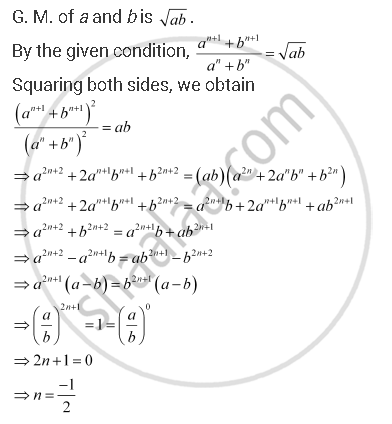Share

# Find the Value Of N So That (A^(N+1) + B^(N+1))/(A^N + B^N) May Be the Geometric Mean Between A And B. - Mathematics

#### Question

Find the value of n so that  (a^(n+1) + b^(n+1))/(a^n + b^n) may be the geometric mean between a and b.

#### SolutionIs there an error in this question or solution?
Find the Value Of N So That (A^(N+1) + B^(N+1))/(A^N + B^N) May Be the Geometric Mean Between A And B. Concept: Geometric Progression (G. P.).